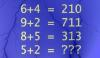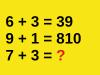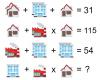BRAIN TEASERSBrain Teasers User Profile

# Stefana Dodevska

rank
458
points
4
See full ranking list
short ranking list
 456 kalikota praveen 4 457 lotus jangid 4 458 Stefana Dodevska 4 459 Prakash Rao 4 460 PRAMOD UIKEY 4
 Mathematical Puzzle: IF 6+4=... Mathematical Puzzle: IF 6+4=210, 9+2=711 and 8+5=313 THEN 5+2=?Mathematical Puzzle: IF 6+3=... Mathematical Puzzle: IF 6+3=39, 9+1=810 and 6+5=111 THEN 7+3=?Can you replace the question mark with a number? MATH PUZZLE: Can you replace the question mark with a number?